## Overview

This guide shows you how to convert a neural network from any framework into an implementation on an Arm Cortex-M-based device, using the Arm CMSIS-NN library.

Popular frameworks, for example TensorFlow, PyTorch, and Caffe, are designed differently. This means that there is not a unified method for converting neural networks for a range of applications across all of these frameworks to run on Arm Cortex-M.

Neural networks are still implemented with floating point numbers. Because CMSIS-NN targets embedded devices, it focuses on fixed-point arithmetic. This means that a neural network cannot simply be reused. Instead, it needs to be converted to a fixed-point format that will run on a Cortex-M device.

CMSIS-NN provides a unified target for conversion. This guide discusses the key challenges that you face when converting a network with CMSIS-NN from any application, and how to approach and overcome those challenges.

At the end of this guide, you will understand the issues involved with the conversion, and how to implement the network with CMSIS-NN by developing efficient and accurate code.

## Before you begin

To aid your understanding of concepts in this guide, you can download and familiarize yourself with these tools:

• CMSIS. You will use two main areas:
• CMSIS-NN library: Read CMSIS-NN: Efficient Neural Network Kernels for Arm Cortex-M for a detailed description of CMSIS-NN.
• CMSIS-DSP, a collection of optimized DSP kernels which can be useful in the context of neural networks for:
• Computing the inputs of a neural network (smart features)
• Implementing new neural network layers
• An ML framework on which you will code, train and analyze your network. This guide can be run on any framework.
• Python with NumPy for the few short code examples. We use Python 3.6.0 and NumPy 1.11.3, but other versions should work, too.
• Keyword spotting patterns. This guide uses the keyword spotting example, but the same steps apply to any other network. Read Hello Edge: Keyword Spotting on Microcontrollers for more information on the keyword spotting

A possible network for the keyword spotting is described in the following diagram. We refer to the keyword spotting network because it is the example that is used most in Arm materials. We use this network at the end of this guide when we demonstrate a user interface with speech recognition.

The user needs to setup a network, because the goal of this document is to convert a network into a CMSIS-NN implementation. The dimensions in the right column are the dimensions of the output of each layer.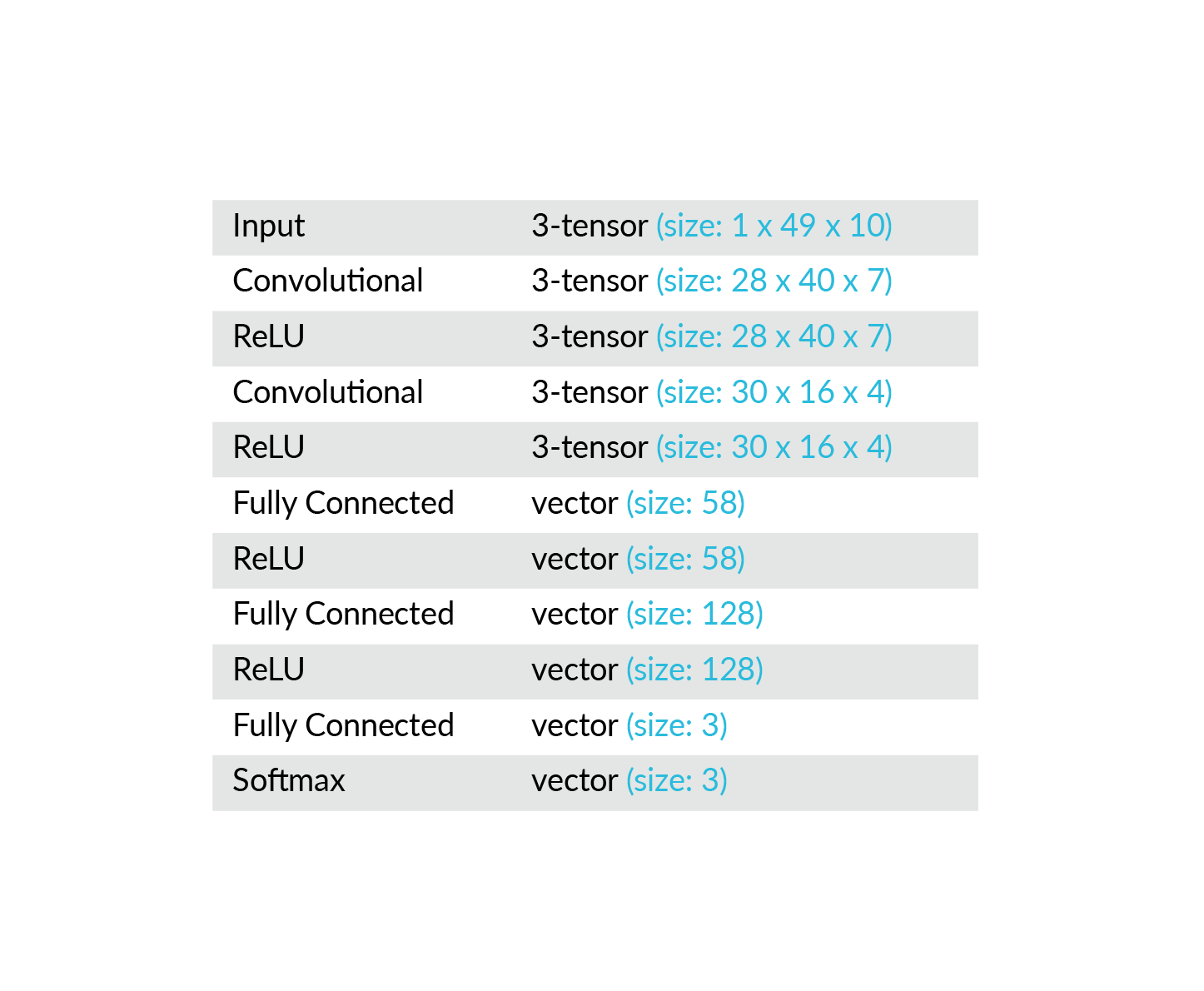If you want to use another network to apply the method described in this guide, then you will need to be sure that CMSIS-NN supports the layers that are used by your network.

## Check the supported layers

CMSIS-NN only supports a small number of layers. The network that you want to convert should contain only layers that are supported by CMSIS-NN. If a layer is not directly supported by CMSIS-NN, that layer should be translatable into an equivalent combination of CMSIS-NN layers and CMSIS-DSP functions. Otherwise, it will not be possible to convert the network into a CMSIS-NN implementation.

For instance, there is no LSTM layer implementation in CMSIS-NN. But a LSTM layer can be expressed with CMSIS-NN and CMSIS-DSP using:

• Fully connected layers (CMSIS-NN)
• Sigmoid and hyperbolic tangent activations (CMSIS-NN)
• Elementwise vector product (CMSIS-DSP)

The CMSIS-NN library contains an example of implementation of a GRU layer with CMSIS-NN and CMSIS-DSP functions. You can look at this example to see how other layers could be expressed. The GRU example is in CMSIS/NN/arm_nn_examples/gru.

## Compare the ML framework and CMSIS-NN data layouts

The terminology used in this guide is a bit different from what is used in mathematics. In this guide:

• A 1D tensor is named vector.
• A 2D tensor is named matrix.
• A > 2D tensor is named tensor.

When we talk about dimensions in this guide, we are referring to the dimensions of the shape of a tensor. We are not referring to the dimensions of a vector space.

The layout of tensors may follow a different convention in the ML framework compared to CMSIS-NN. For example, the elements in a matrix can be arranged in row or column order in memory. For a general tensor, there is a greater choice of orderings that correspond to permutations of the dimensions.

The weights of the layer must be reordered to be used with CMSIS-NN if:

• The ML framework and the CMSIS-NN orderings are different
• The layer is a convolutional layer or a fully connected layer
• The input of the layer is a matrix or tensor
##### Example with a fully connected layer

As an example, let’s look at the common case in which a fully connected layer is following a convolutional layer. In that case, the input of the fully connected layer is a tensor. Let’s also assume that the dimensions of this tensor are {2,3,4}. (These are the minimum dimensions that we can use as an example if we want all dimensions to be different).

Let’s name this input Torg. It has 24 elements, as you can see in the following tensor: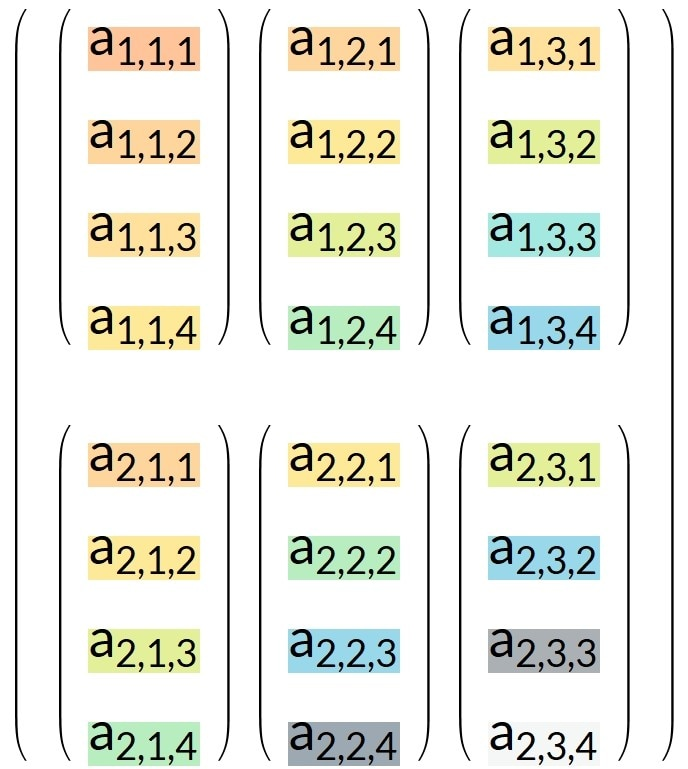This tensor can also be represented as a 3D object, as you can see in the following image: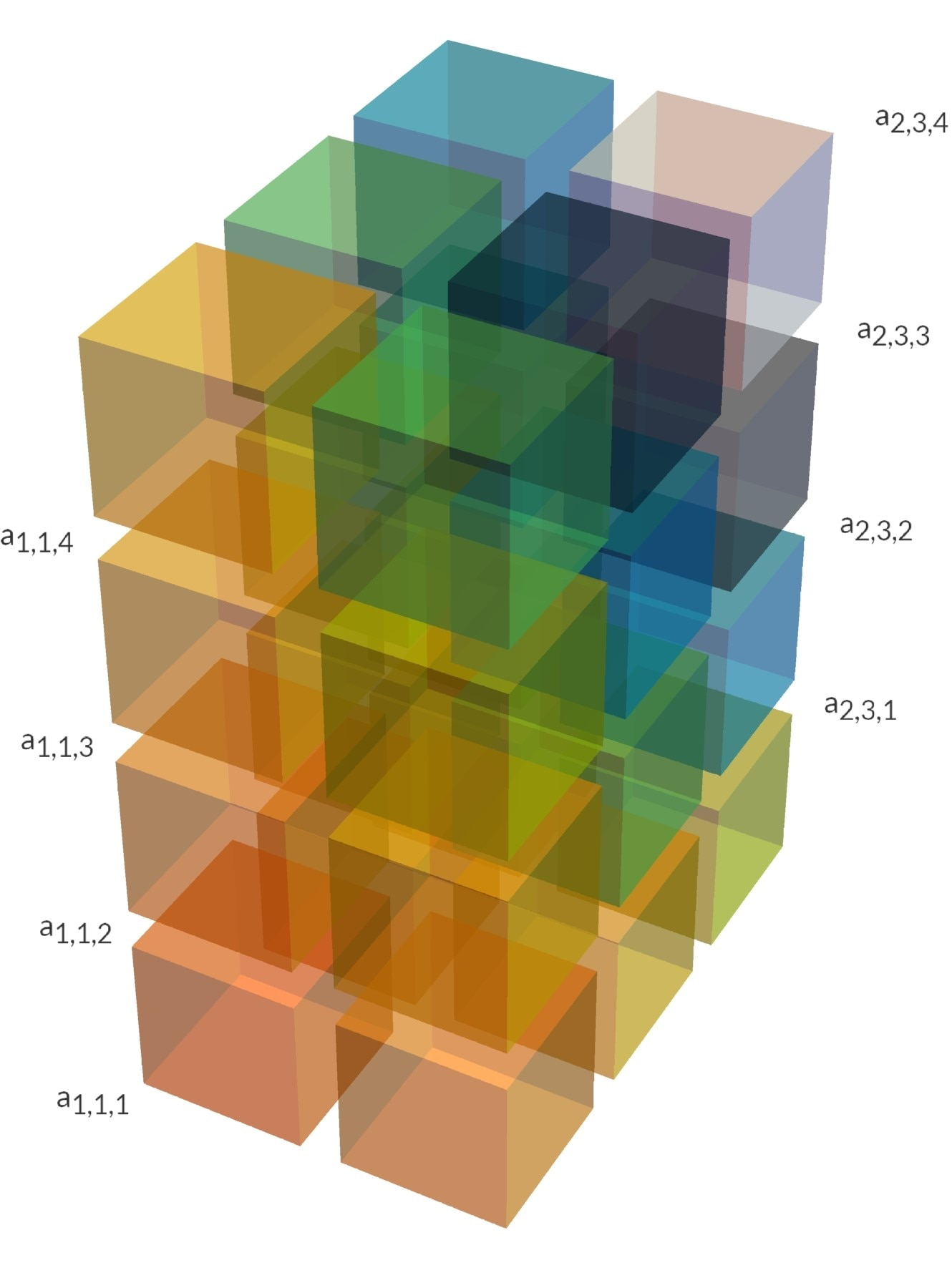The fully connected layer is using a vector as input. It is a flattened version of tensor Torg. Let’s name this flattened tensor FTorg. It is a vector and has also 24 elements, as shown in the following table: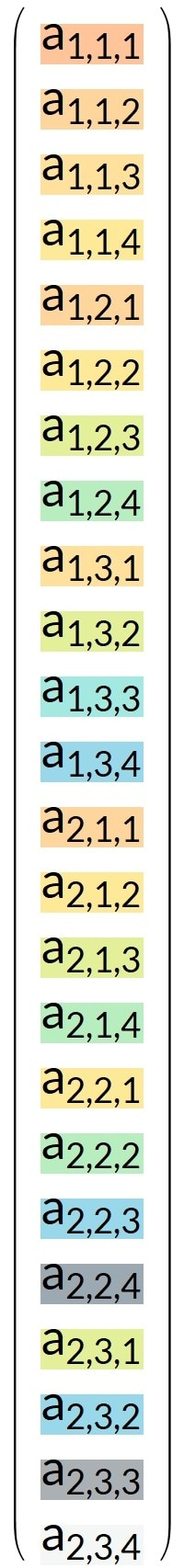Depending on which framework you use, this flattening may, or may not, be modeled in the original ML framework with a flattening layer. Some frameworks do the flattening automatically.

The weight matrix for the original fully connected layer is Worg. Let’s assume that the output of this fully connected layer has two elements, so that the weight matrix has the dimensions {2,24}. The matrix is transforming a vector of 24 elements and into a vector of two elements.

The weight matrix Worg is displayed in the following table: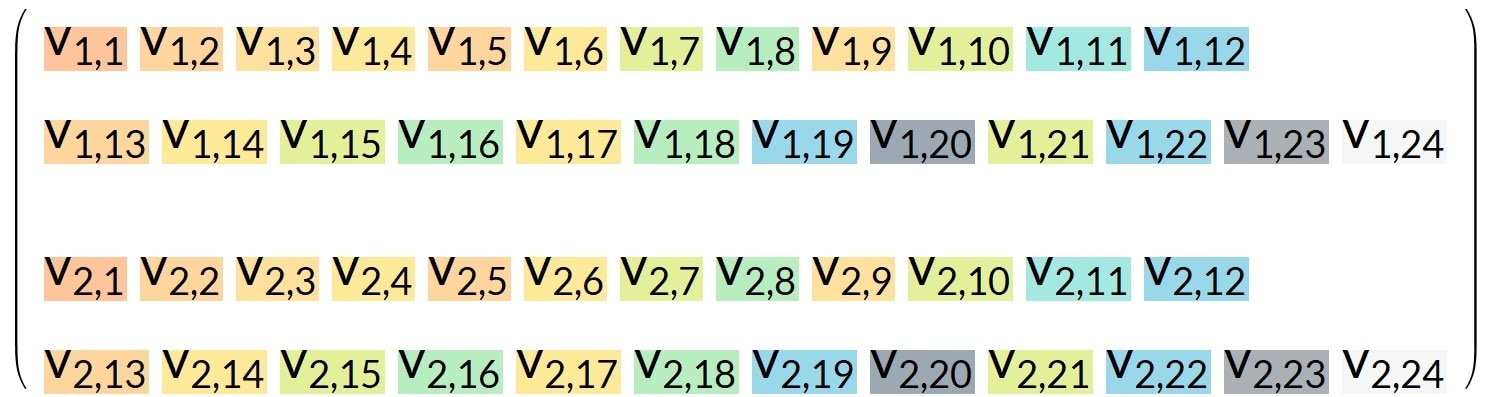The purpose of the fully-connected layer is to compute the matrix product:

Worg. FTorg,

In this example, the fully connected layer output is this column of two values: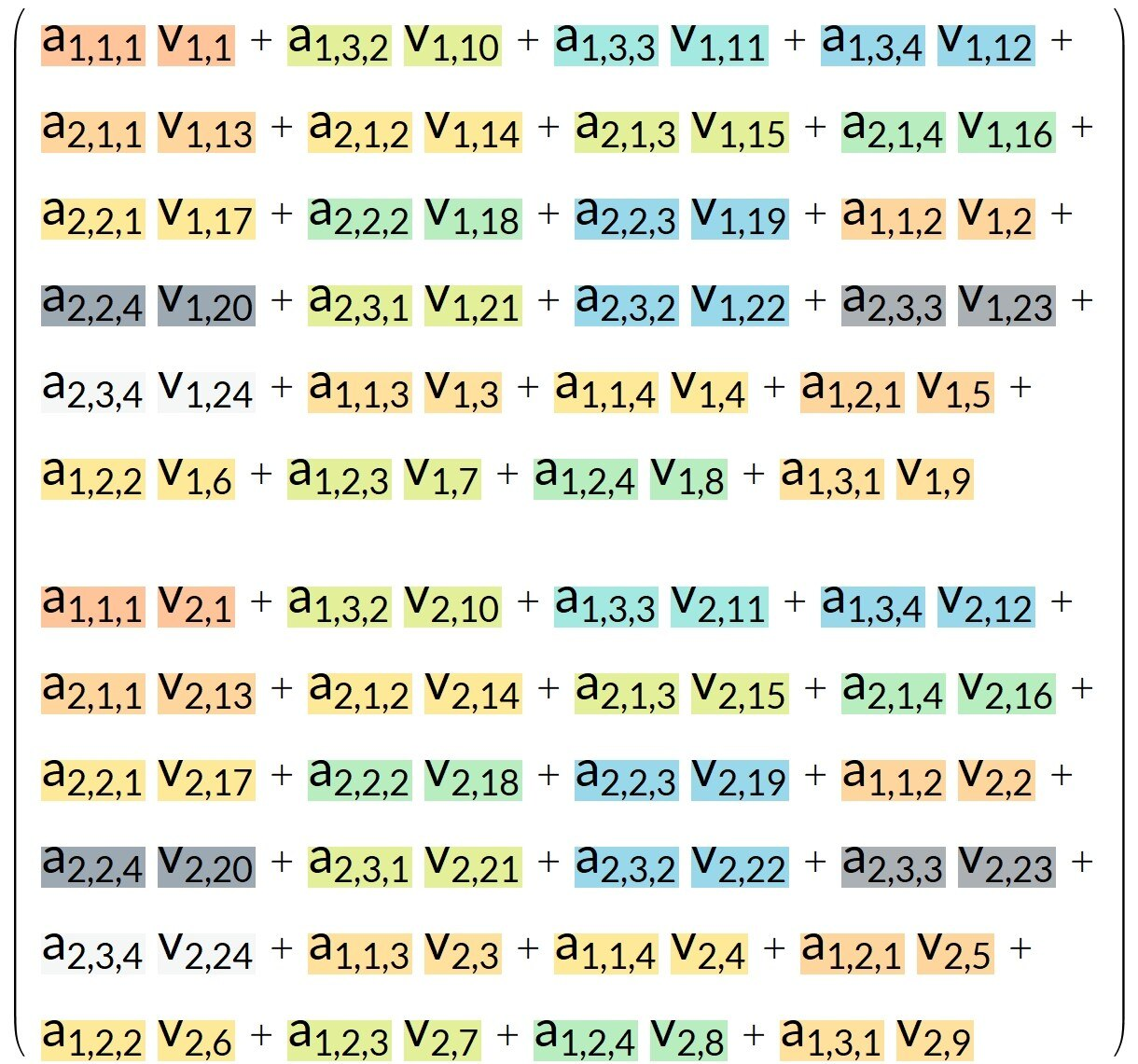Let’s look at an example in which:

• CMSIS-NN orders the data differently to how it is ordered in the original ML framework
• the input tensor is the transposed tensor Tnew with dimensions {4,3,2}

This example is shown in the following table: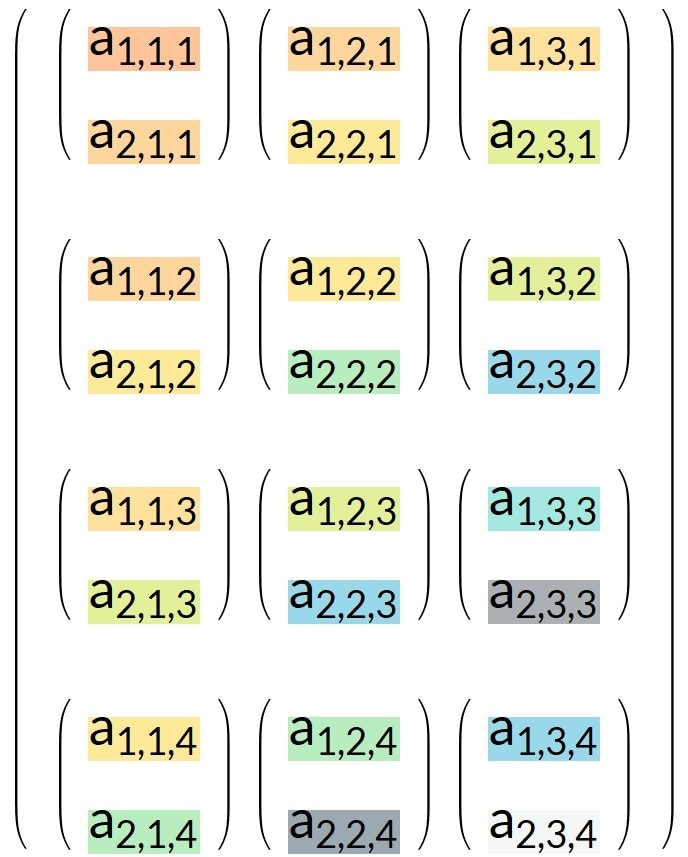This transposed tensor can also be represented as a 3D object, as you can see in this image: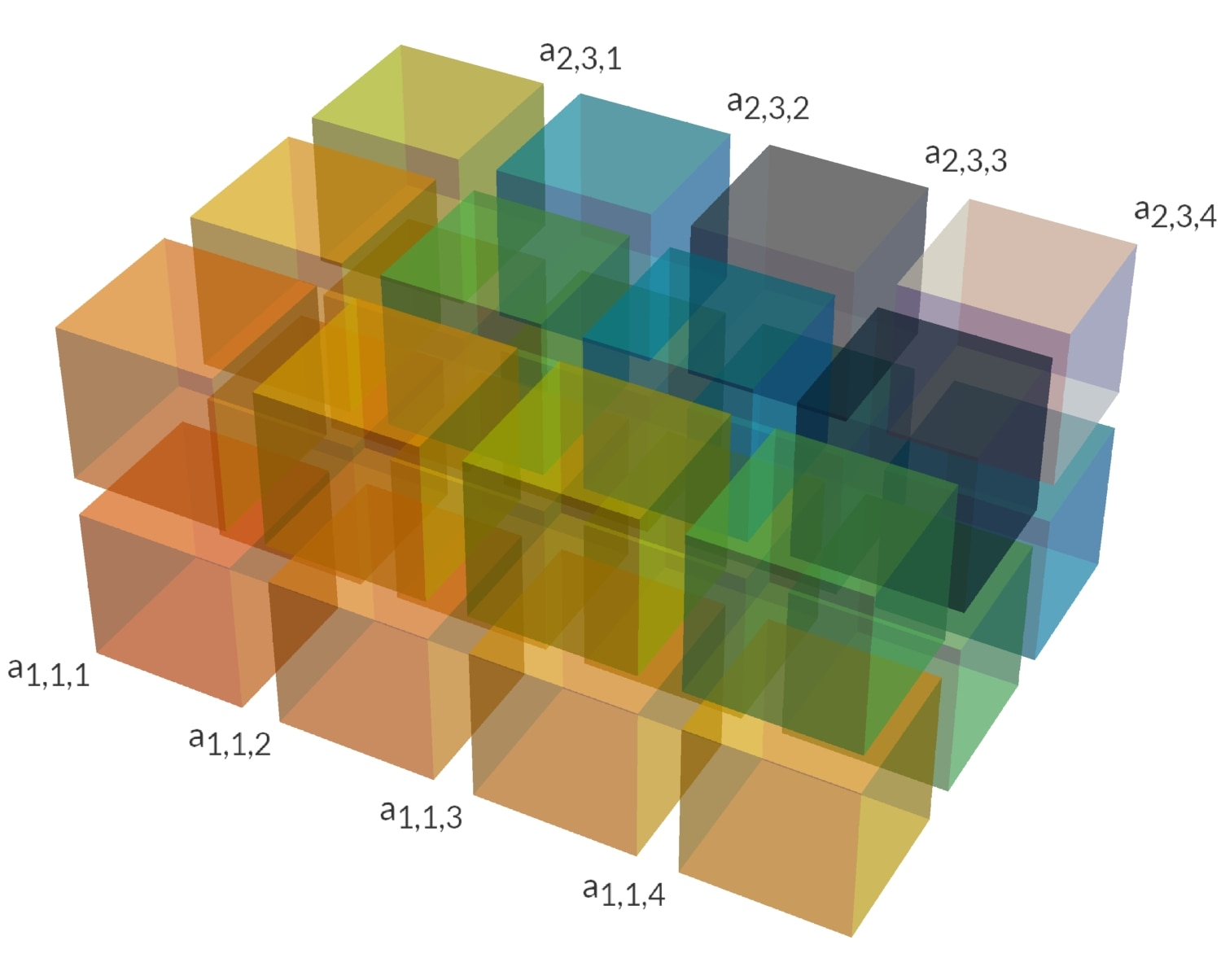Here is an animation showing the transposition operation: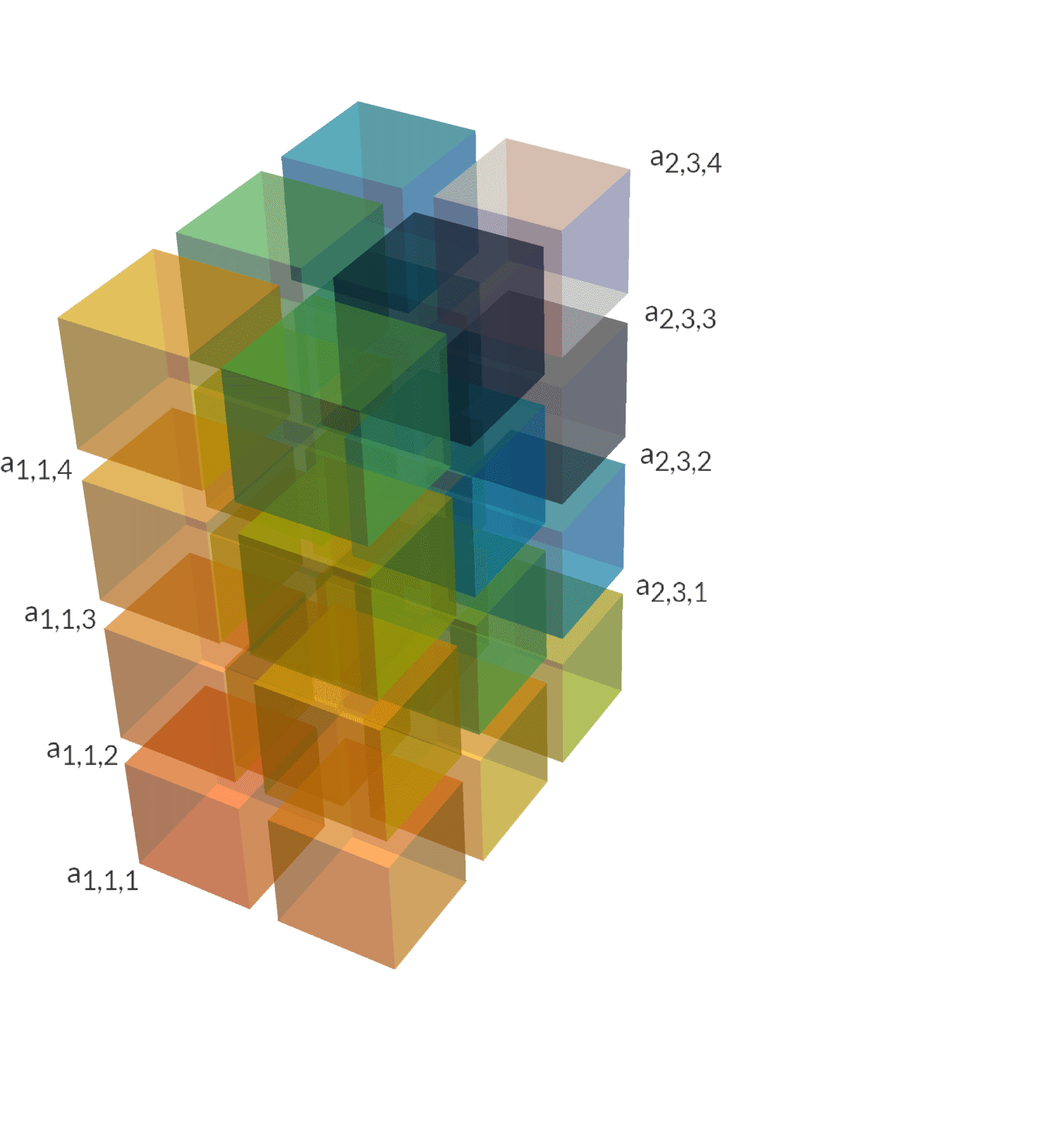In NumPy, if torg is the original tensor, then the conversion can be done with:

tnew = torg.transpose(2,1,0)

The flattened vector FTnew corresponding to tnew is displayed below: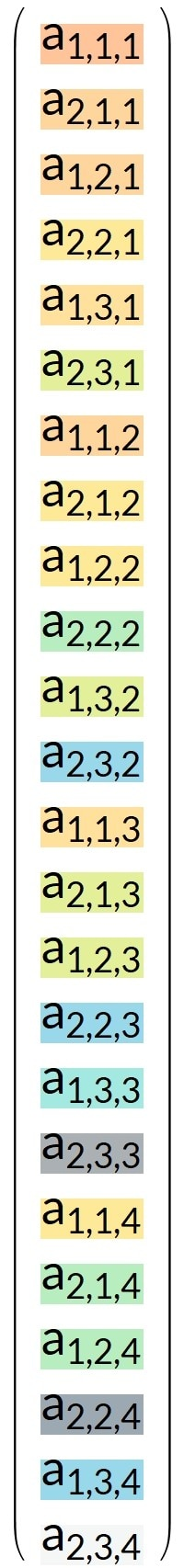In NumPy, this flattened tensor can be computed with:

tnew.reshape(24)

What should the reordering of the weight matrix be, so that the output of the fully connected layer is still the same?

Let’s name this new reordered weight matrix Wnew. For display purposes, here is the transposed version of Wnew.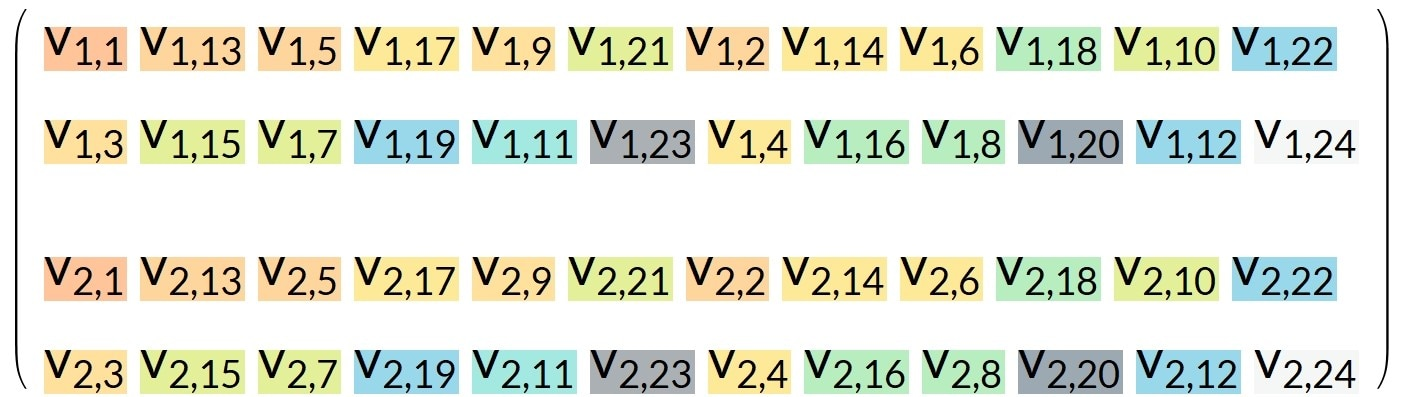Here is an animation showing the weight reordering: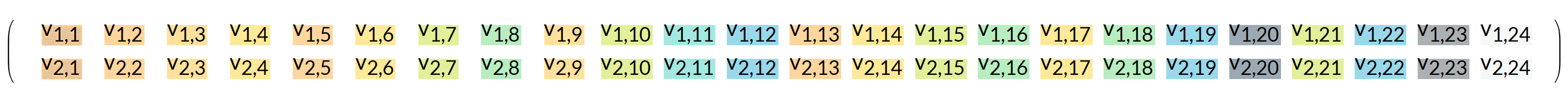If we compute the new matrix product, we must get the same output because the CMSIS-NN implementation must behave as the original ML layer:

Wnew . FTnew == Worg . FTorg

In NumPy, if the weight matrix before reordering is named worg, and the weight matrix after reordering is wnew, then we can get the reordering of the weights with:

wnew = worg.reshape(2,2,3,4).transpose(0,3,2,1).reshape(2,24)

Then we can check that the output computed with reordered weights and reordered input is the same. In NumPy, it can be checked that:

np.dot(worg ,torg.reshape(24)) should be equal to np.dot(wnew , tnew.reshape(24))

The NumPy code for reordering can be decomposed like this:

• The beginning of the line, worg.reshape(2,2,3,4), is transforming the matrix into a tensor. The matrix has dimensions {2,24}. In this example, 2 is the output dimension of the fully connected layer. The input vector of length 24 is the flattened version of a tensor of dimensions {2,3,4} and we need to recover those dimensions. The numbers in the reshape command can be easily related to the input and output dimensions. The command can be written in pseudo-code as: reshape(output dimension, input tensor dimensions).
• Once the weight matrix has been converted into a tensor, it is possible to permute its dimensions. This permutation should correspond to the permutation applied on the input when going from the ML framework to CMSIS-NN. This permutation is the transpose(0,3,2,1) part of the above code. The output dimension is not permuted hence a first 0. Only the tensor dimensions are permuted, and in this example the permutation is a reversal.
• Finally, the tensor is converted back into a {2,24} matrix.
##### Example with a convolutional layer

In case CMSIS-NN is using a different ordering convention than the ML framework, then the procedure to reorder the weights for a convolutional layer is the same as the one for a fully connected layer, but simpler. This is because you do not need to convert a matrix into a tensor and back. The permutation of dimensions can be directly applied to the tensor.

In CMSIS-NN, the input and output of convolutional layers have the following dimensions:

{in channel, x dimension, y dimension}

From the C code for the inner loop of the convolutional layer in CMSIS-NN, we can read that the weight tensor has dimensions:

{in channel, x kernel, y kernel, out channel}

Now, assume your ML framework is working with inputs of dimensions:

{y dimension, x dimension, in channel}

And assume that the weight tensor of the ML framework has corresponding dimensions:

{y kernel, x kernel, in channel, out channel}

The dimensions must be reordered so that the new weight tensor has the dimensions in the order that CMSIS-NN expects:

{in channel, x kernel, y kernel, out channel}

And it is clearly visible that it is just a reverse of the three first dimensions.

In NumPy, it could be done with:

tnew = torg.transpose(2,1,0,3)

The first three dimensions are reversed. The last one is not changed.

Because any necessary reordering is not simple and depends on the details of your ML framework, test the final reordering with a float version of CMSIS-NN. There is no official float version, but it is easy to create one, as we will show

Extract the q15 version from the reference implementations used for the unitary testing of CMSIS-NN. The reference implementations can be found in CMSIS/NN/NN_Lib_Tests/Ref_Implementations.

In the reference q15 implementation:

• q15 must be replaced by float
• The bias and out shifts must be removed
• Saturation functions must be removed

The result will be a float version that can be used to validate the weight reordering, if any reordering is needed.

Note: In CMSIS-NN, there is another reordering of the weights which can be applied for performance purpose, only when the _opt versions of the fully connected functions are used. This weight reordering is explained in the optimization section of this guide.

At the end of this section of the guide, you should know what reordering is required for each layer and how to do it. This reordering will be needed when it is time to dump the coefficients for use with CMSIS-NN.

## Quantization

Quantization of a network is a difficult problem. This is because, when switching from floating point to fixed point arithmetic, truncation noise and saturation effects are introduced.

There are two ways to address the problem of quantizing a network:

• Training with a quantized network
• Quantizing an existing network

The first solution should to give better results since the network is trained with the truncation noise and the saturation effects. But it is also more complex than the second method.

Some ML frameworks provide operators to model quantization and saturation. For example, TensorFlow includes quantization operators like fake_quant_with_min_max_vars and fake_quant_with_min_max_vars_gradient.

Other frameworks may have similar operators. If the network is rewritten with those operators in the right places, which some automated tool can do, then:

• The network can be trained with quantization effects
• Statistics like min/max can be generated for the input/output of each layer

However, these framework operators are not totally equivalent to the fixed-point implementations. This is because these are float implementations and focus on the input and output but not on the internal computations of the fixed-point kernels. Those internal computations can saturate, or suffer from sign inversion issues, and they are generating a different computation noise than a float implementation.

If a full equivalence with CMSIS-NN is required, you should extend your framework used with kernels that behave like the CMSIS-NN ones. However, the inference and back-propagation would have no reason to require the same accuracy, and you may need a version of the kernels with more fixed-point accuracy for the back-propagation.

Therefore, we can see that training with a quantized network:

• Is more complex
• Is framework dependent
• May not model all effects of the fixed-point implementation

For the reasons stated above, we implement the second strategy, quantizing an existing network, because it is simpler and framework independent.

With the second strategy, quantizing the weights and biases is simple. This is because the values are known, so that it is easy to find the fixed-point format as soon as the word size is chosen. For CMSIS-NN, the word size can either be 8 bits or 16 bits.

For the activation values, for example input and output of layers, quantizing is more difficult and is described in the following section.

## Compute activation statistics

To quantize the input/output of the network layers, you need to know the range of values. This means that you will need to evaluate the network on several patterns and record the values at the input and output of each layer. Ideally, the full set of training patterns should be used.

You probably do not want to keep all the values, because it is a lot of data if you evaluate the network on all the training patterns.

In that case, the simplest solution would be to keep the min and the max values. However, you may want to have more information about the distribution of values to experiment with several quantization scheme later.

So another possibility is to compute a histogram for each input and output, and upgrade it each time the network is evaluated on a new training pattern. Because you are only interested in the number of bits that are required to represent the values, the bins of the histogram can be based upon powers of two.

In the images below, you can see some histograms of the activations of some layers of the KWS network. In each image, the left shows the input of a layer and the output is on the right. The scale is logarithmic.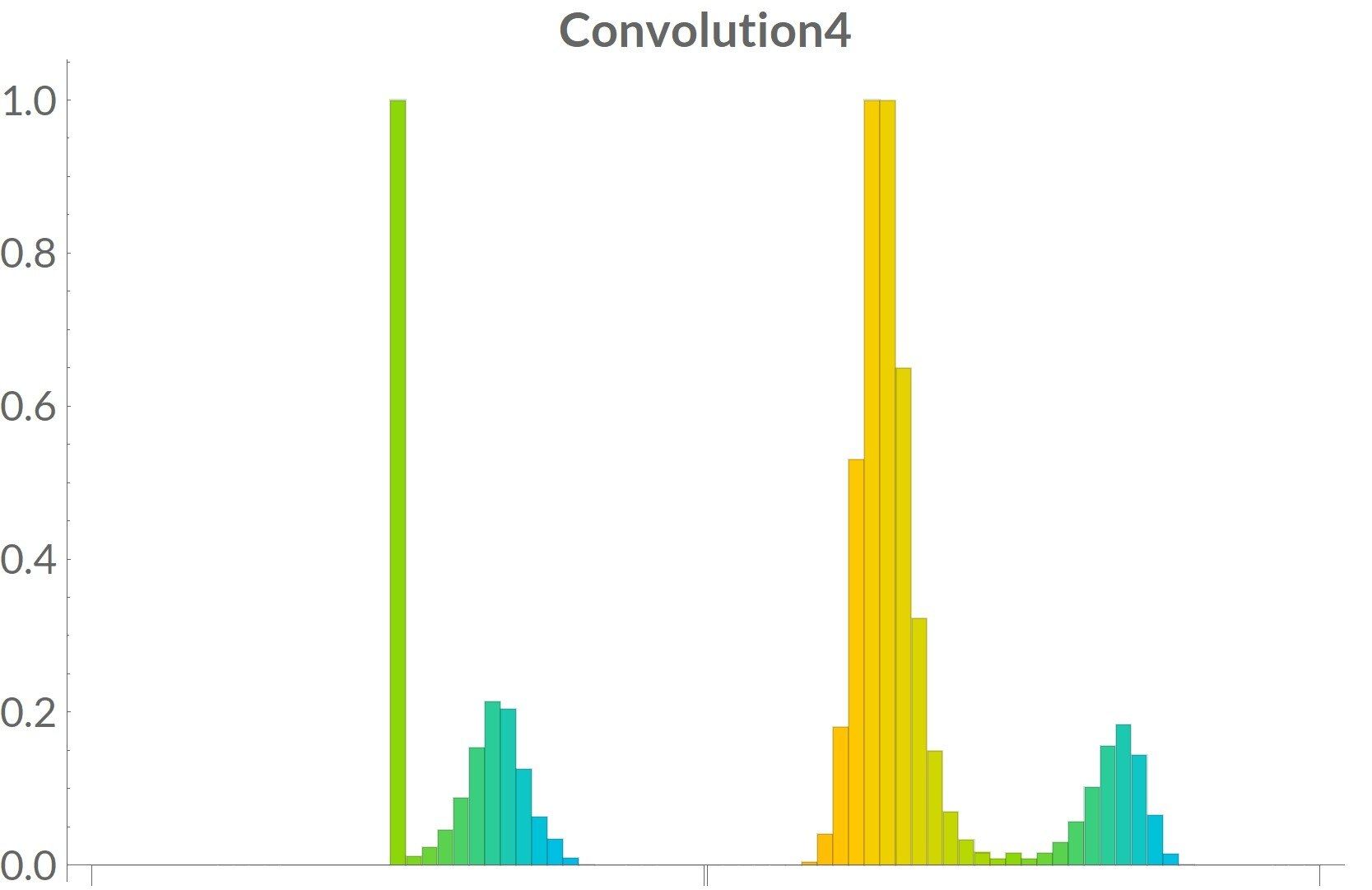Convolution4 is showing the input of a convolution layer on the left part of the picture. Since this input is also the output of a previous ReLU layer then it is highly asymmetric which is visible on the graph.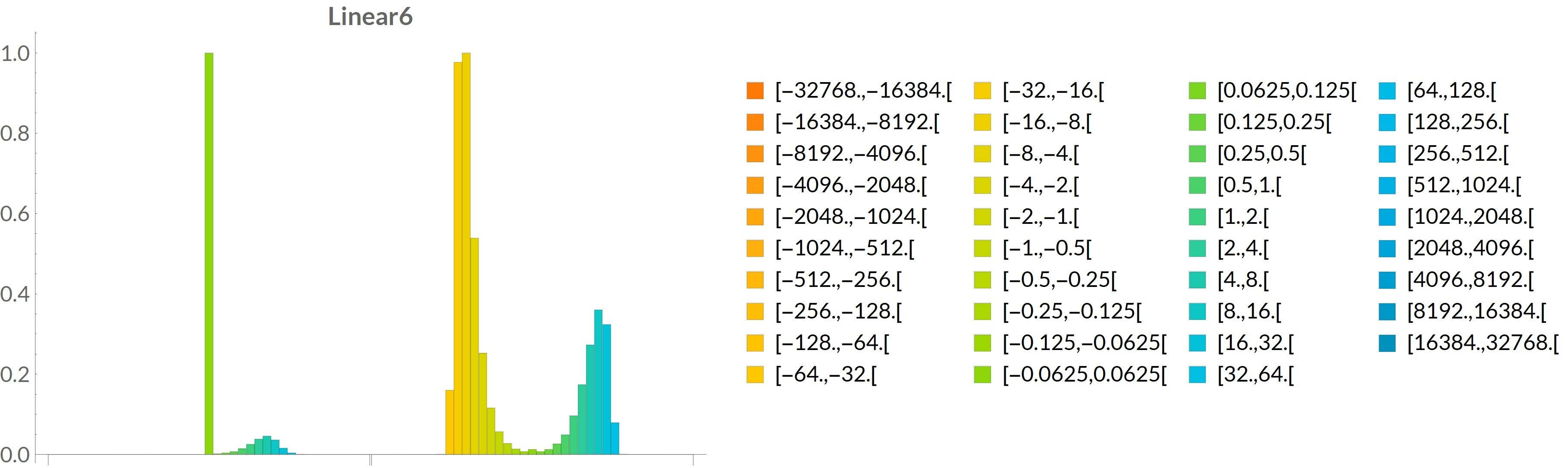The horizontal scale is the same for all pictures. As we can see on this picture, power of two values were used as the bounds of the different histogram bins.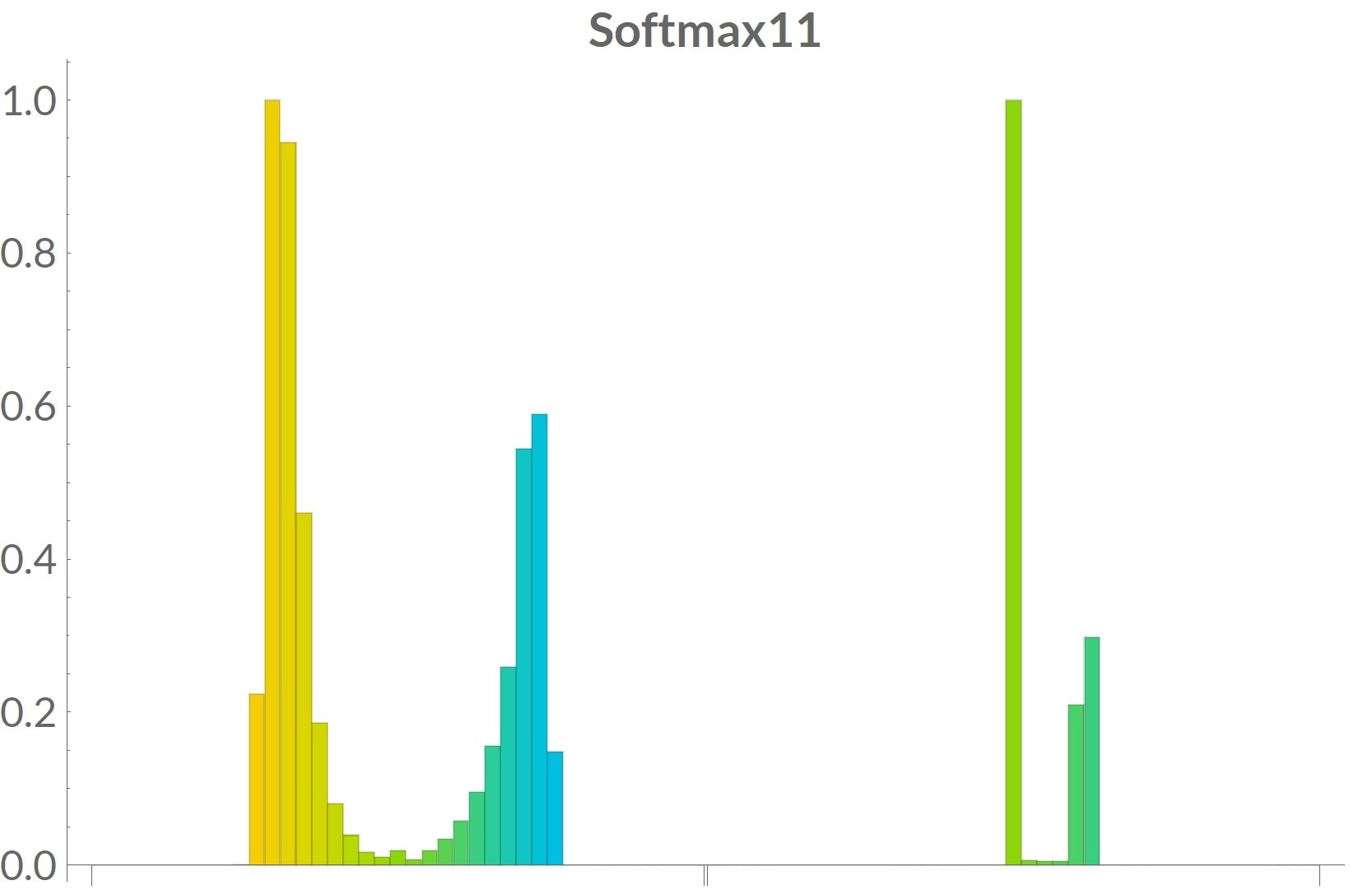## Choose a quantization scheme

From previous histograms, we can see that the values are often concentrated. This means that several strategies may be tested:

• Using the full range of values, quantization based upon the min and max
• Focusing only on the most likely values, quantization using the range of values making 80% of the histogram, or any other percent you may want to try
• More complex schemes to detect and remove outliers from this distribution of values

You need to choose one scheme and then do the quantization based on this choice. You’ll have to experiment with several schemes, because results can vary depending on the network and training patterns.

For some networks, you may want to use the full range and base the quantization on the min and max. For other networks, you may get better results by using a smaller range than the min and max and removing outliers from the distribution.

A higher range means that you decrease the probability of internal saturations or sign inversions, but because the statistics are only on input and output and not intermediate computations, this problem can still occur. But having a higher range also means that you have fewer bits for the fractional part and less accuracy. Testing is needed to find the right trade-off.

There is also another factor to take into consideration: the chosen word size. The number of fractional bits you can represent depends on the range of values and the word size.

Most CMSIS-NN functions have an 8-bit version and a 16-bit version. Choosing one of these versions be part of the definition of the quantization scheme. This choice must be the same for all layers, or conversions between 8-bit and 16-bit would have to be introduced between layers.

## Compute the layer Q-formats

Once you have statistics for each layer and a choice of a quantization scheme, you can deduce the Q-format for the inputs and outputs of the layers.

The Q-formats computed from the statistics and word size are based upon the assumption that the output format can be chosen independently from the input one. It is not possible for all layers.

Some layers impose constraints on the output format. For instance, the Q-format of the output of a max pool is the same as its input format, because of how the algorithm is implemented in CMSIS-NN.

Therefore, you cannot freely choose the output format for a max pool layer even if, according to the statistics, a better choice may be possible.

It is only for fully connected layers and convolutional layers that it is possible to choose the output format independently from the input format by shifting the biases and the output values.

At this step you need to choose a Q-format for each input and output. You need to consider:

• How the layers are connected
• Which layers are allowing to customize the output format

Start with the network input Q-format based on the statistics of the training patterns. If the input layer is a fully connected or convolutional one, then define the output Q-format based upon the output statistics. Otherwise, the output Q-format is computed from the input format and the nature of the layer.

Iterate the procedure one layer after another. At the end you should have a list of Q-format for all the input and output of the layers.

This procedure is summarized in the following diagram: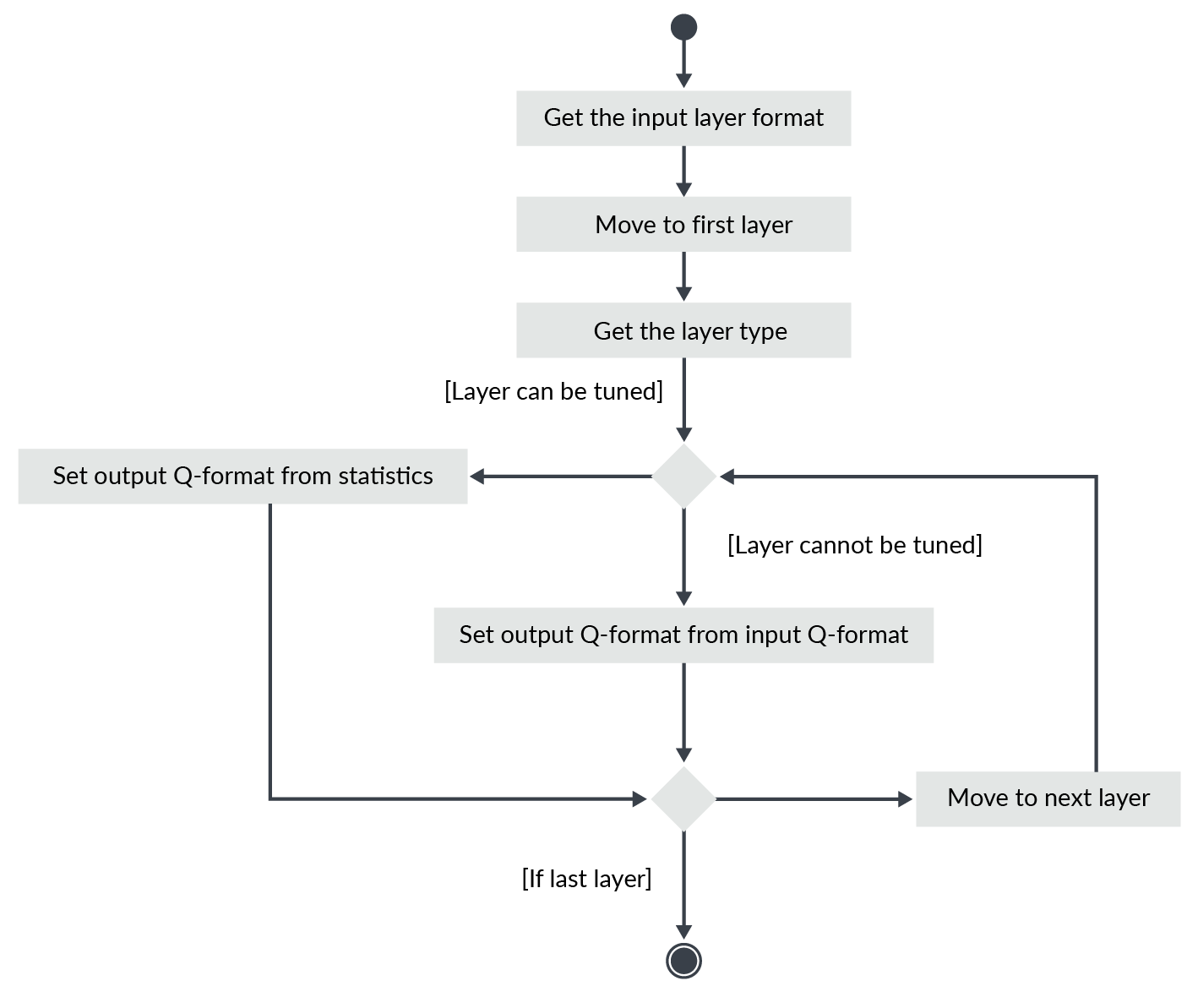## Compute the layer shifts

As explained in Compute the layer Q-formats, it is only for the fully connected and convolutional layers than the output Q-format can be defined independently from the input format.

Once you know the Q-format of the input and output of the fully connected and convolutional layers, then you can compute the bias shift and out shift. Those shifts are used to ensure that the result of the layer computation has the requested output Q-format.

If fi is the number of fractional bits for the input, fo for the output, fw for the weight and fb for the biases then:

The bias shift is : (fi + fw) - fb

The out shift is : (fi + fw) - fo

This quantization approach will not show whether problems might occur during the computations. For this reason, it is important to test the final behavior of the quantized network.

If you really want to know what is happening in the internal computations, like some possible saturations or sign inversions, the inference should be modified to keep track of the dynamic of the internal computations.

This modification is easy to do it in any language in which basic operations like addition and multiplication can be overloaded. But you may need to write your own inference implementation and use the CMSIS-NN implementation with overloaded basic operations.

## Generate the CMSIS-NN implementation

Once the Q-formats are known and the bias and out shifts are known, you are ready to generate the quantized coefficients and code for use with CMSIS-NN.

Start with the first layer. If it is a fully connected or convolutional layer, quantize the reordered weights and quantize the biases. Then dump those coefficients into C arrays. Dump a function call to the layer function using the bias, and the out shift which were computed for this layer and the parameters of the layer.

If it is another kind of layer, just dump a function call to the layer function using the correct parameters for the layers.

Parameters include, for example, stride, padding, kernel size, input dimensions, and output dimensions.

The process is summarized in the following diagram: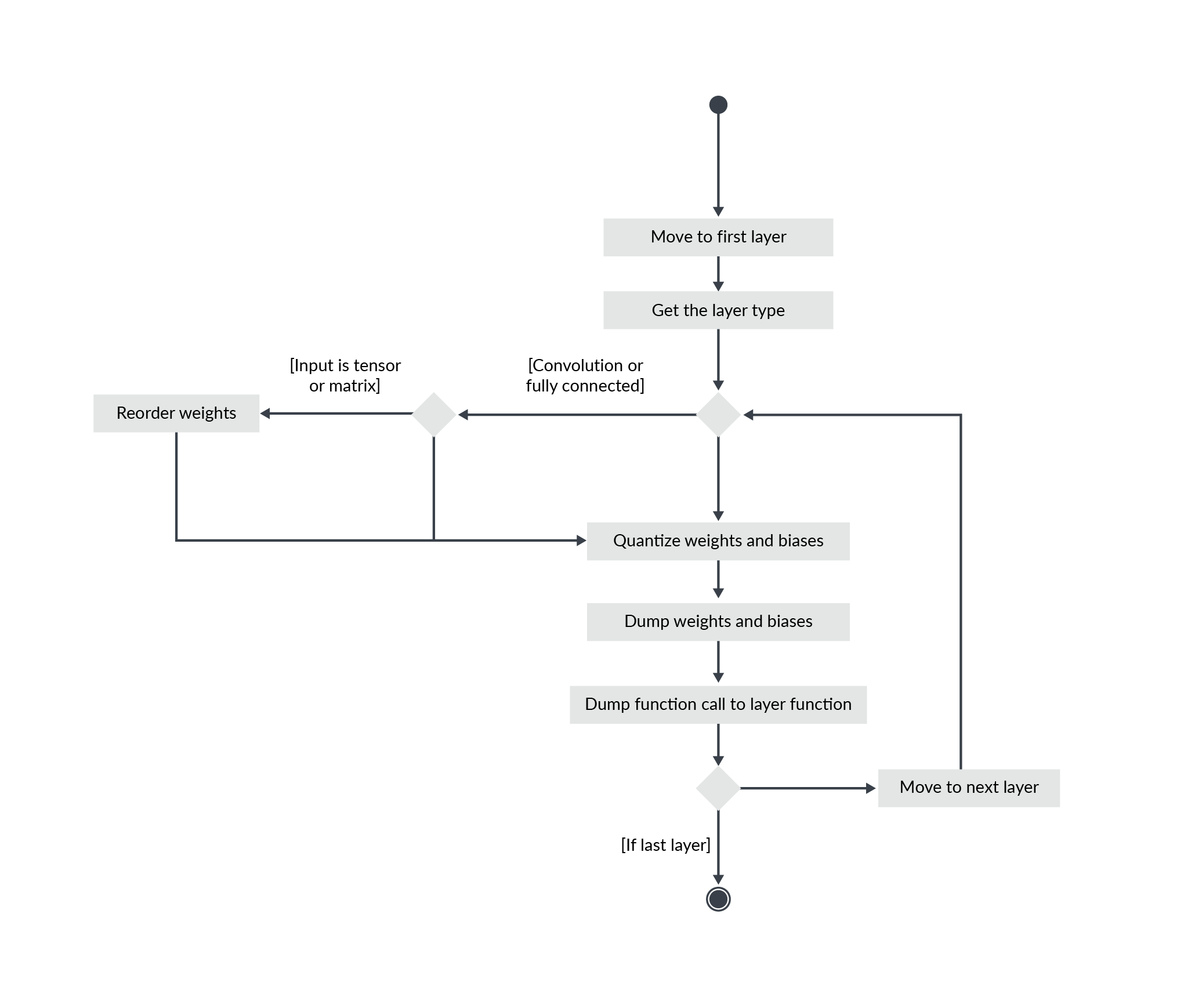Each layer requires several buffers for its processing:

• Input and output buffers, which can be the same if the layer is doing in place modifications
• Temporary buffers for q7 versions of some functions

All of those buffers must also be allocated when generating the C code.

Finally, you need to look at the input and output of the network.

The input has no reason to be in the Q-format expected by the network. The input is in the format defined by your sensors and your pre-processing code.

The input buffer of the network should be initialized with values in the right Q-format. This means that some pre-processing may have to be added if the input is generated on the device in a format which is not adapted.

If the format conversion of the input adds too much overhead for the application, then the input Q-format may be chosen differently to avoid this pre-processing step. In that case, this constraint should be considered when computing the Q-formats for all the layers.

This different format may not be the one giving the best result, so testing is required, as explained in the Testing this result section of this guide.

The output may have to be converted, too, if the code following the network is expecting values with a different format than the format generated by the network. If the latest layer of the network is not tunable, because there is no shift parameter, then a post-processing step may be required to do this conversion.

## Test the result

It is very important that you test the final result. Because of effects present in the fixed-point implementation but not modeled in the ML framework, you cannot rely only on the performance estimations done in the framework.

The accuracy of the final version depends on the details of the quantization. Different quantization schemes can give very different performances. So, it is important that you test the final implementation and that you experiment with different quantization schemes.

The testing should be done on the full set of test patterns, which should be different from the training and validation patterns.

It is possible to communicate with a CMSIS-NN implementation running on a board. But the set of test patterns is expected to be quite big. This means that it may be faster to use a CMSIS-NN implementation running on your desktop to do the final testing.

Choose a development environment in which it is easy to call C code. With such an environment, a CMSIS-NN implementation can be used directly for testing.

CMSIS-NN compiled in CM0 mode, which is ARM_MATH_DSP undefined in the C code, only requires implementation of _SSAT and _USAT. C implementations of those intrinsics are available in the CMSIS library in CMSIS/Core/Include.

With a little work, it is easy to get CMSIS-NN running on a desktop or laptop and use it directly.

Once the CMSIS-NN implementation of the network can be used directly, you can compute some metrics by using your test patterns. Then you should compare those results with the metrics for the original network.

It is also good to be able to play with the network to get an intuitive understanding of how it behaves. It does not replace quantitative metrics, but it is a good complement.

In the following graphics, you can see a comparison of the reference network for keyword spotting, running in an ML framework, and a q15 CMSIS-NN implementation. In this UI, the user can play with different noise levels and different words and see how the final CMSIS-NN implementation is behaving compared to the original implementation in the ML framework.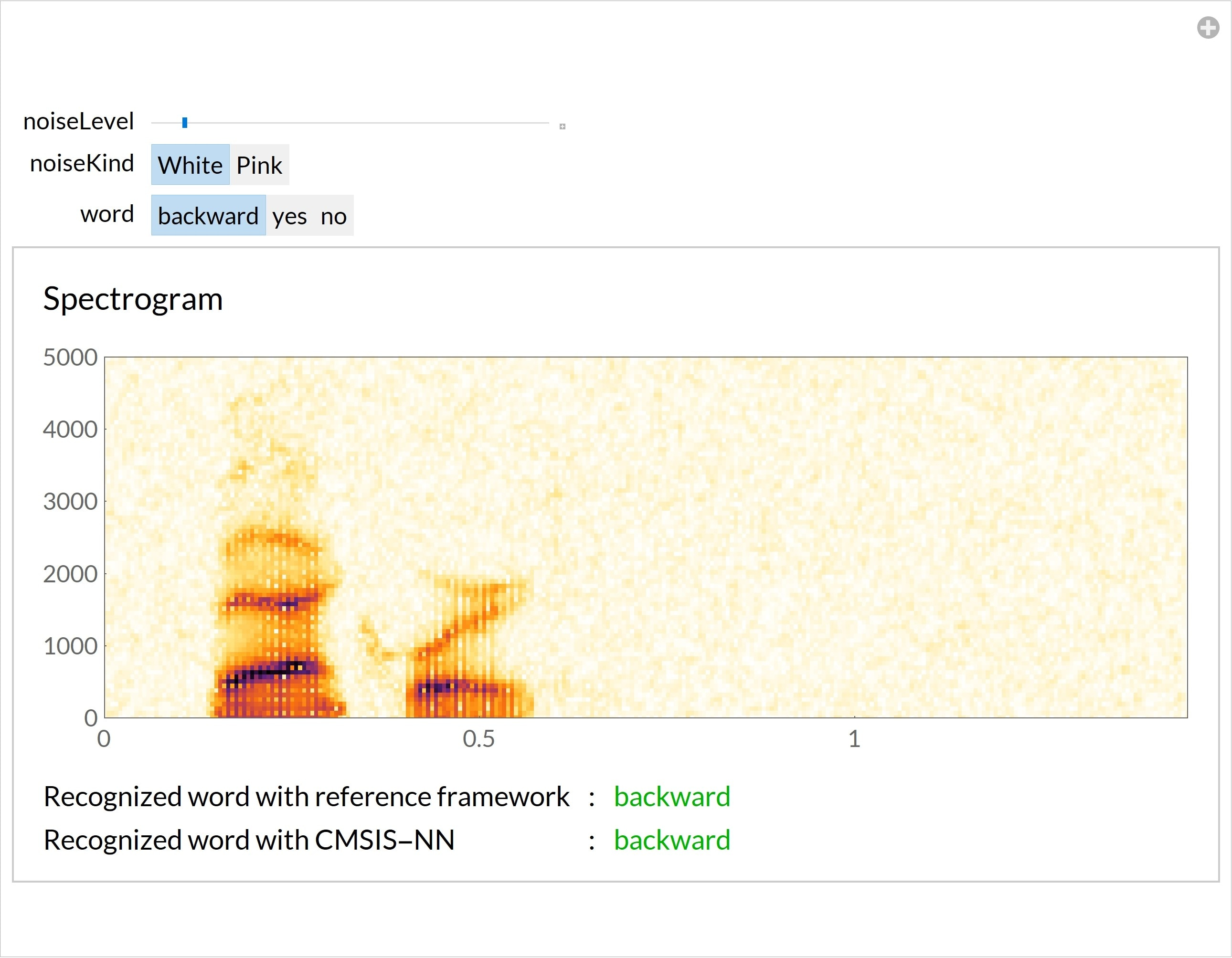## Optimize the final implementation

In CMSIS-NN, there are several versions of the kernels depending on the values of the layer dimensions. For instance, there is a square version of functions for convolutional layers.

There are also some specific _opt versions of the fully connected layers which require some additional weight reordering. CMSIS-NN: Efficient Neural Network Kernels for Arm Cortex-M CPUs describes this.

The final implementation should use the most efficient version of each layer.

Also, often several buffers in the network have the same size. When those buffers are not used at the same time, the memory should be reused to minimize the number of buffers required for the full network.

## Summary

To convert any network to CMSIS-NN, the following key steps must be followed:

• Check which layers are the supported layers.
• If some layers are not supported, you should try to replace them with an equivalent combination of CMSIS-NN and CMSIS-DSP functions.
• Check the data layout convention.
• If the conventions are different, some weight reordering will be needed and should be tested with a float version of CMSIS-NN.
• Compute activation statistics.
• Choose the statistics that must be computed and use enough input patterns to generate those statistics.
• Choose a quantization scheme.
• Choose a word size.
• Define a method to select a fixed-point format from the computed statistics and the word size.
• Compute the layer Q-formats.
• Compute input and output Q-format for each layer based upon the quantization scheme and the layer constraints. Bias and out shifts for fully connected and convolutional layers should be known after this step.
• Generate the CMSIS-NN implementation:
• Dump reordered and quantized coefficients for weights.
• Dump quantized biases.
• Dump function calls to CMSIS-NN functions.
• Allocate needed buffers.
• Test the final fixed-point version.
• Ideally, test on the same set of test patterns as the original version.
• If the final fixed-point version is not good enough, you may need to go back to a previous step, for example changing the quantization method or changing the network.
• It is good to be able to play with the network to get a feeling for how it is behaving. It is completing the quantitative data about its performances.
• Optimize the final CMSIS-NN code.
• Use the most efficient version of each layer function.
• Minimize the memory usage by reusing buffers as much as possible.

## Next steps

This guide was built using a keyword spotting network as an example. But a keyword spotting network cannot work alone. The network is using the output of a Mel-Frequency Cepstral Coefficient (MFCC) as input. The MFCC is computing the features used by the network for the recognition.

In theory, one could imagine a network using the audio samples as input instead of the MFCC. Such a network would be trained to learn how to extract features from the audio samples.

It would not be efficient to do it like that on an embedded system. A network computing features equivalent to an MFCC would be bigger (in memory and cycles) than just using an optimized MFCC implementation.

For embedded systems, it is useful, for performance reasons, to do some signal processing on the input signal before using the neural network. Hence, in a full solution, CMSIS-DSP should also be used.

For the same reasons, the final layer of a network may be replaced by other classifiers when it allows to decrease the memory usage and number of cycles of the full solution.